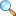Unit 07: Linear Equations and Inequalities

These notes are send by Mr. Malik Faisal Rafiq. We are very thankful to him for sending these notes.

Linear Equations: A linear equation is one unknown variable $x$ is an equation of the form $ax+b=0$, where $a,b\in \mathbb{R}$ and $a\neq 0$.

Radical Equations: When the variable in an equation occurs under a radical, the equation is called radical equation.

Equation Involving Absolute Value: The absolute value of real number $a$ denoted by $|a|$ is defined as $$|a|=\left\{\begin{matrix} a & \text{if } a\geq 0, \\ -a & \text{if } a<0. \end{matrix}\right.$$ e.g. $|6|=6$, $|0|=0$, $|-3|=3$.

Defining inequalities: Let $a,b$ be real numbers. Then $a$ is greater than $b$ if the difference $a-b>0$ and we denote this order relation by the inequality $a>b$. An equivalent statement is that $b$ is less than $a$, symbolized by $b<a$.

Solutions

• Exercise 7.1 |View Online | Download PDF (178.7 KiB, 5112 downloads)
• Exercise 7.2 |View Online | Download PDF (101.93 KiB, 3569 downloads)
• Exercise 7.3 |View Online | Download PDF (115.41 KiB, 3935 downloads)
• matric/9th_science/unit07